#### 期刊菜单

Numerical Study on the Effect of Temperature on the Mechanical Properties of Aluminum Foam Sandwich Shells

Abstract: In this paper, numerical simulation methods are used to study the influence of temperature on the mechanical properties of aluminum foam sandwich shells. First, a finite element model of the alu-minum foam sandwich shells has established, and an impact load was applied. The simulation re-sults were compared with the experimental results to verify the accuracy of the finite element model. Secondly, the influence of temperature on the energy absorption and deformation of the aluminum foam sandwich shells is studied. The results show that at temperatures ranging from −50˚C to 300˚C, the energy absorption of the sandwich shells does not change significantly as the temperature increases. However, the deformation of the center point of the back panel and the structure’s overall deformation will increase with the increase of temperature, and the impact re-sistance of the entire structure will decrease. Finally, the failure mode of the aluminum foam sand-wich shells is analyzed. The results show that under the projectile’s impact, the sandwich shell mainly undergoes shear failure at −50˚C, 25˚C, and 300˚C. The upper and lower panels of the sand-wich shell are mainly the shear failure, and the aluminum foam core layer is the failure by compac-tion and collapse at the same time as the shearing failure occurs.

1. 前言

2. 有限元模型验证

2.1. 材料属性Figure 1. Stress-strain curve of aluminum foamTable 2. Material thermal parameters

2.2. 有限元模型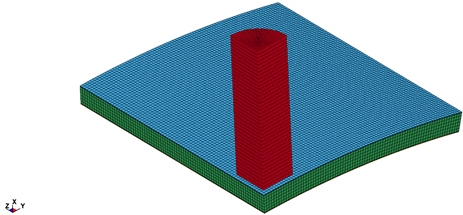Figure 2. Finite element calculation model

2.3. 模型验证

( $\stackrel{¯}{I}=\frac{I}{{A}_{c}\left(2{\rho }_{f}h+{\rho }_{c}c\right)\sqrt{{\sigma }_{f}/{\rho }_{f}}}$，其中 ${A}_{c}$ 为子弹作用区域的面积， ${\rho }_{f}$${\rho }_{c}$ 分别为面板和泡沫铝的密度， ${\sigma }_{f}$

3. 温度对泡沫铝夹芯壳力学性能的影响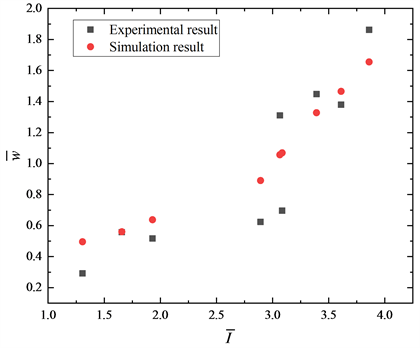Figure 3. Simulation results and experimental results of the center point of the back panel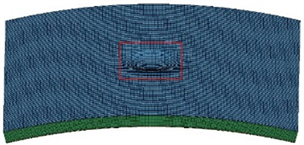Figure 4. Deformation of aluminum foam sandwich shell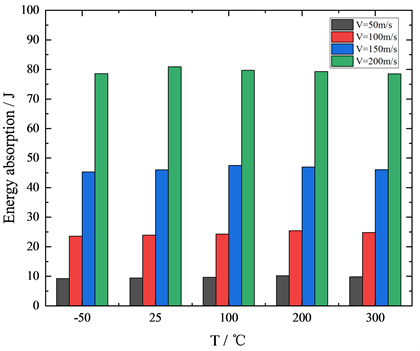Figure 5. Energy absorption of aluminum foam sandwich shell at different temperature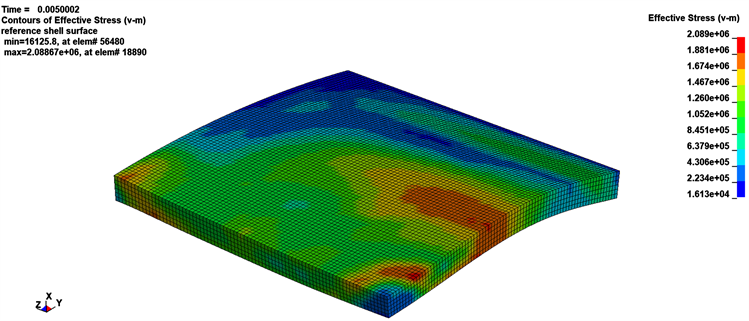(a) V = 50 m/s, T = 300℃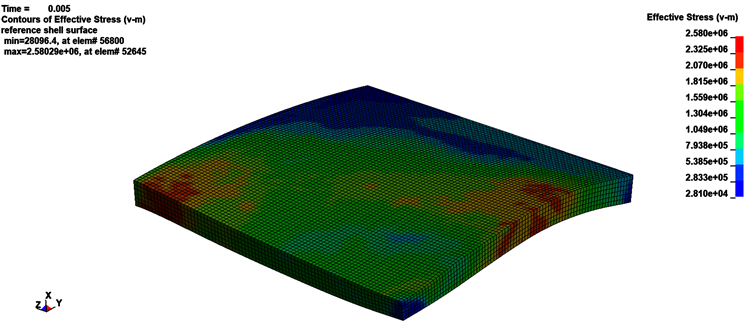(b) V = 100 m/s, T = 300℃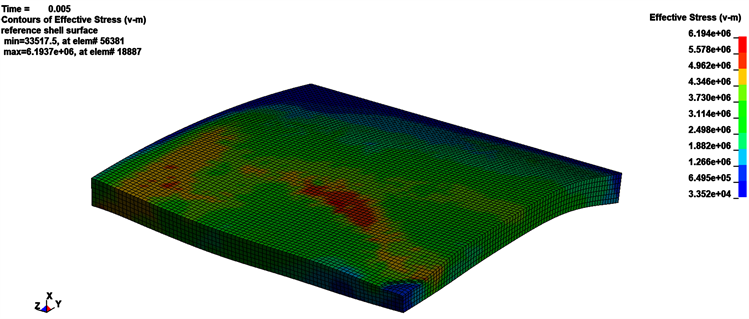(c) V = 150 m/s, T = 200℃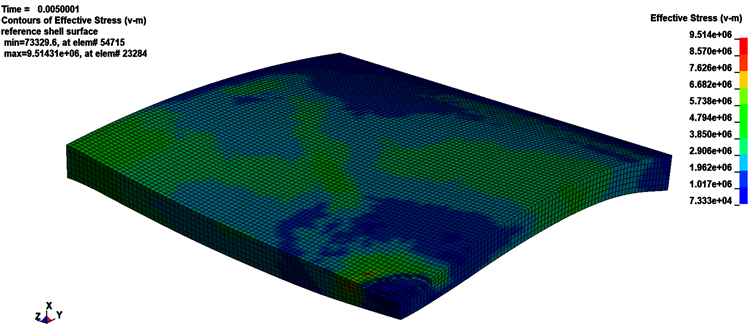(d) V = 200 m/s, T = 100℃

Figure 6. Deformation diagram of aluminum foam at different projectile velocities and different temperatures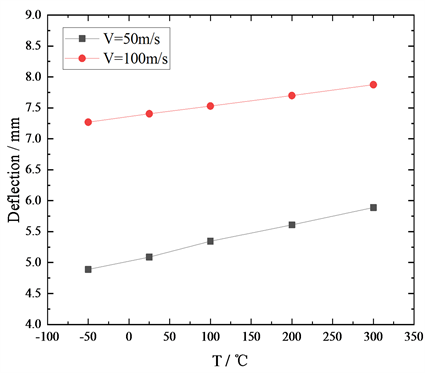Figure 7. Deformation of the center point of the back panel of the aluminum foam sandwich shell at different temperatures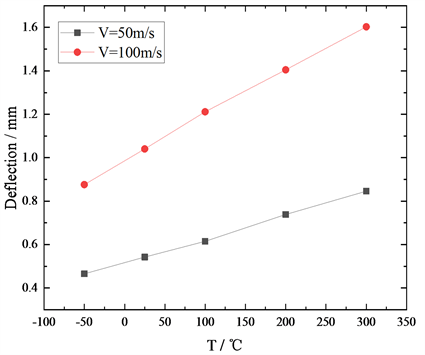Figure 8. Overall deformation of the back panel of the aluminum foam sandwich shell at different temperatures

4. 夹芯壳在不同温度下的破坏形式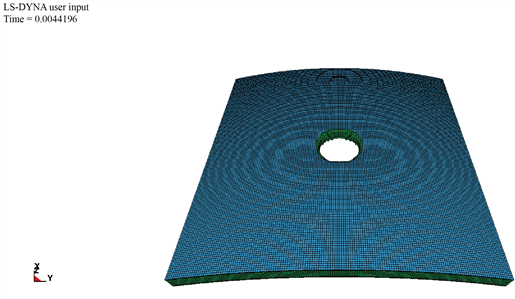(a) T = −50℃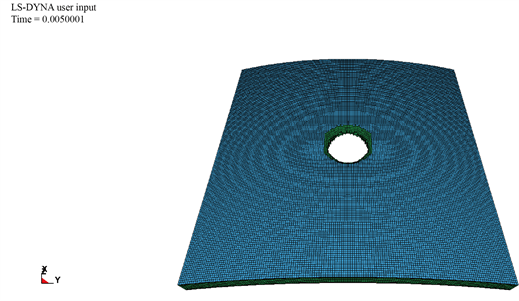(b) T = 25℃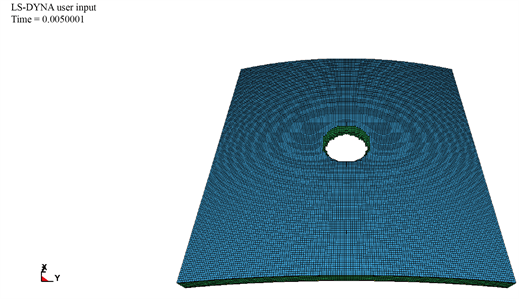(c) T = 300℃

Figure 9. The simulated failure model of the sandwich shell at different temperatures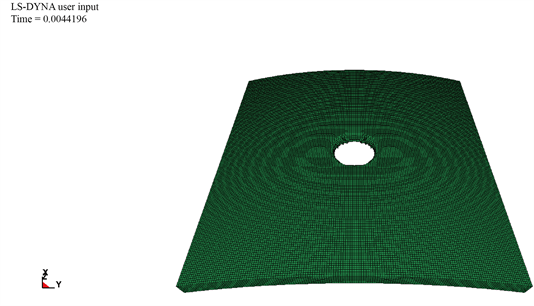(a) T = −50℃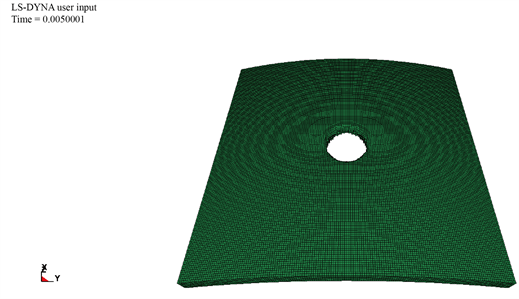(b) T = 25℃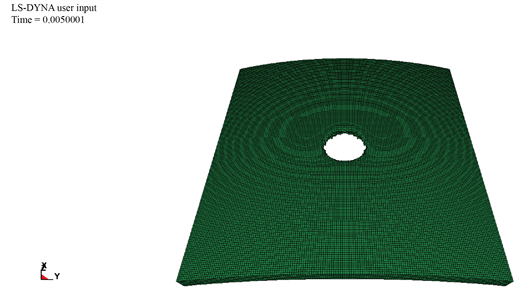(c) T = 300℃

Figure 10. The simulated failure model of the aluminum foam at different temperatures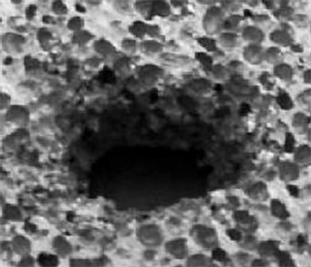Figure 11. The experimental failure model of the aluminum foam core at ambient temperature (25˚C)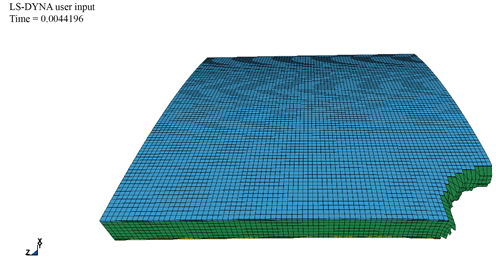(a) T = −50℃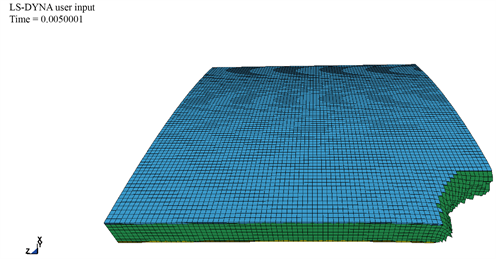(b) T = 25℃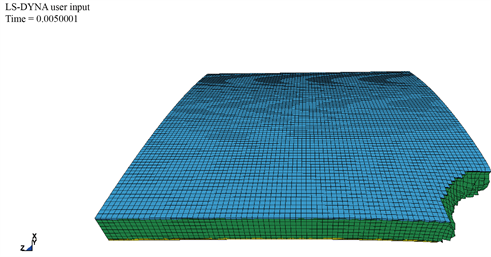(c) T = 300℃

Figure 12. The simulated failure model of the 1/4 model of the sandwich shell

5. 结论